Next: ASTRONOMICAL BACKGROUND RADIATION Up: INFRARED ASTRONOMY FROM SPACE Previous: INTRODUCTION

# INFRARED UNITS

The infrared wavelength range has borrowed units from the surrounding optical and radio (submm) regimes. Both magnitude and flux representations are widely used. For the ISO proposals (scaled) SI units are required.

Flux density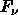of a source is defined as the flux per unit frequency. Flux density is the physical unit used for point sources. The unit of flux density is Wm-2 Hz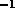. For practical purposes in the infrared it is often convenient to scale this SI unit and use Jansky instead.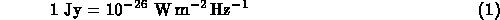For extended objects, the surface brightness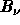is used instead of flux density. Also background emission for e.g. point sources is expressed in brightness units. Brightness is defined as the flux density per unit solid angle. The unit of brightness is WmHzsr, but in practice it is often scaled to MJysr.Flux density of a point source, in particular for a stellar object, is often given in magnitudes m. In the ISO wavelength range these units are mostly used for ground based measurements below 20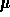m. The conversion to or from magnitudes is performed via zero magnitude flux density, which depends on the wavelength and the photometric system used. Zero magnitude flux densities for ESO infrared filters J through Q are listed in Table 5. Magnitude m of a ground based observation can be converted into flux density with formulaFilter[m] [m] [Jy] J 1.24 0.2 1587 H 1.64 0.3 1074 K 2.18 0.4 653 L' 3.76 0.7 253 M 4.69 0.5 150 N 10.3 5.2 29.4 N8.38 0.8 48.7 N9.67 1.6 34.9 N12.9 3.7 19.7 Q 18.6 5.6 9.5

For spectroscopic measurements, the total flux F of a line is often the most interesting quantity. A flux calibrated spectrum may be presented in the form of flux densityas a function of wavelength. Flux F of a line in interest can be obtained by subtracting an appropriate continuum flux density level of the spectrum before integrating over the entire line in wavelength. The unit of flux is Wm-2.Next: ASTRONOMICAL BACKGROUND RADIATION Up: INFRARED ASTRONOMY FROM SPACE Previous: INTRODUCTION

ISO Science Operations Team
Tue Aug 6 11:04:33 MET DST 1996